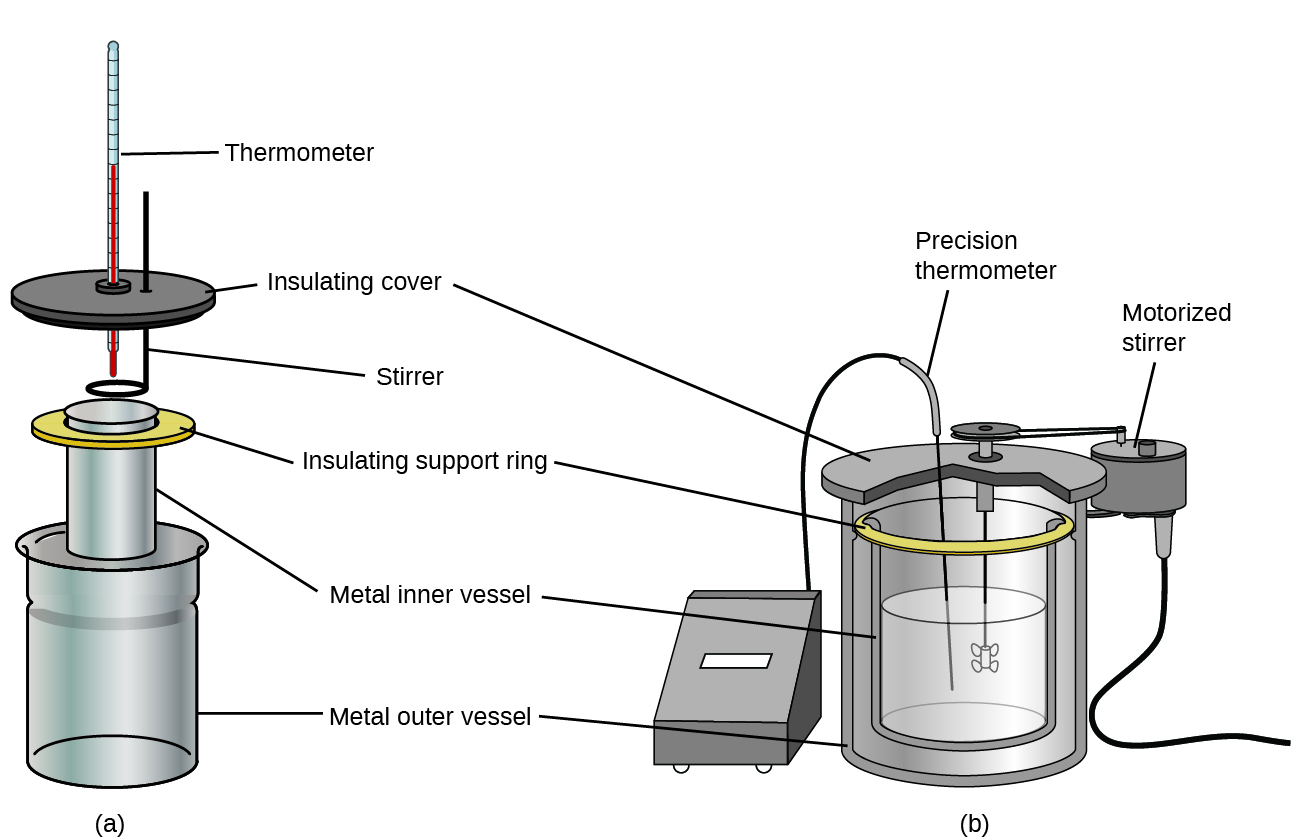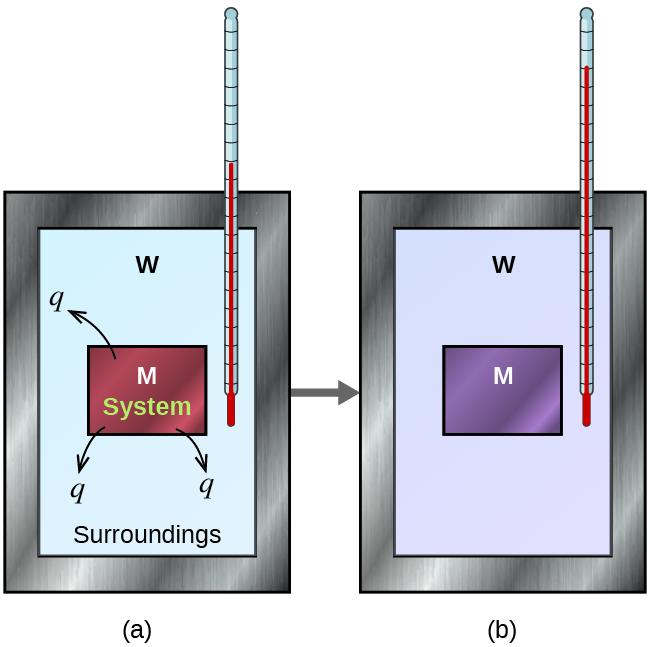# 5.4 Thermochemistry: calorimetry  (Page 2/14)

 Page 2 / 14Commercial solution calorimeters range from (a) simple, inexpensive models for student use to (b) expensive, more accurate models for industry and research.

Before we practice calorimetry problems involving chemical reactions, consider a simpler example that illustrates the core idea behind calorimetry. Suppose we initially have a high-temperature substance, such as a hot piece of metal (M), and a low-temperature substance, such as cool water (W). If we place the metal in the water, heat will flow from M to W. The temperature of M will decrease, and the temperature of W will increase, until the two substances have the same temperature—that is, when they reach thermal equilibrium ( [link] ). If this occurs in a calorimeter, ideally all of this heat transfer occurs between the two substances, with no heat gained or lost by either the calorimeter or the calorimeter’s surroundings. Under these ideal circumstances, the net heat change is zero:

${q}_{\text{substance M}}+{q}_{\text{substance W}}=0$

This relationship can be rearranged to show that the heat gained by substance M is equal to the heat lost by substance W:

${q}_{\text{substance M}}=\text{−}{q}_{\text{substance W}}$

The magnitude of the heat (change) is therefore the same for both substances, and the negative sign merely shows that q substance M and q substance W are opposite in direction of heat flow (gain or loss) but does not indicate the arithmetic sign of either q value (that is determined by whether the matter in question gains or loses heat, per definition). In the specific situation described, q substance M is a negative value and q substance W is positive, since heat is transferred from M to W.In a simple calorimetry process, (a) heat, q , is transferred from the hot metal, M, to the cool water, W, until (b) both are at the same temperature.

## Heat transfer between substances at different temperatures

A 360-g piece of rebar (a steel rod used for reinforcing concrete) is dropped into 425 mL of water at 24.0 °C. The final temperature of the water was measured as 42.7 °C. Calculate the initial temperature of the piece of rebar. Assume the specific heat of steel is approximately the same as that for iron ( [link] ), and that all heat transfer occurs between the rebar and the water (there is no heat exchange with the surroundings).

## Solution

The temperature of the water increases from 24.0 °C to 42.7 °C, so the water absorbs heat. That heat came from the piece of rebar, which initially was at a higher temperature. Assuming that all heat transfer was between the rebar and the water, with no heat “lost” to the surroundings, then heat given off by rebar = −heat taken in by water , or:

${q}_{\text{rebar}}=\text{−}{q}_{\text{water}}$

Since we know how heat is related to other measurable quantities, we have:

${\left(c\phantom{\rule{0.2em}{0ex}}×\phantom{\rule{0.2em}{0ex}}m\phantom{\rule{0.2em}{0ex}}×\phantom{\rule{0.2em}{0ex}}\text{Δ}\text{T}\right)}_{\text{rebar}}={-\left(c\phantom{\rule{0.2em}{0ex}}×\phantom{\rule{0.2em}{0ex}}m\phantom{\rule{0.2em}{0ex}}×\phantom{\rule{0.2em}{0ex}}\text{Δ}\text{T}\right)}_{\text{water}}$

Letting f = final and i = initial, in expanded form, this becomes:

${c}_{\text{rebar}}\phantom{\rule{0.2em}{0ex}}×\phantom{\rule{0.2em}{0ex}}{m}_{\text{rebar}}\phantom{\rule{0.2em}{0ex}}×\phantom{\rule{0.2em}{0ex}}\left({T}_{\text{f,rebar}}-{T}_{\text{i,rebar}}\right)=\text{−}{c}_{\text{water}}\phantom{\rule{0.2em}{0ex}}×\phantom{\rule{0.2em}{0ex}}{m}_{\text{water}}\phantom{\rule{0.2em}{0ex}}×\phantom{\rule{0.2em}{0ex}}\left({T}_{\text{f,water}}-{T}_{\text{i,water}}\right)$

The density of water is 1.0 g/mL, so 425 mL of water = 425 g. Noting that the final temperature of both the rebar and water is 42.7 °C, substituting known values yields:

where we get a research paper on Nano chemistry....?
nanopartical of organic/inorganic / physical chemistry , pdf / thesis / review
Ali
what are the products of Nano chemistry?
There are lots of products of nano chemistry... Like nano coatings.....carbon fiber.. And lots of others..
learn
Even nanotechnology is pretty much all about chemistry... Its the chemistry on quantum or atomic level
learn
da
no nanotechnology is also a part of physics and maths it requires angle formulas and some pressure regarding concepts
Bhagvanji
hey
Giriraj
Preparation and Applications of Nanomaterial for Drug Delivery
revolt
da
Application of nanotechnology in medicine
what is variations in raman spectra for nanomaterials
ya I also want to know the raman spectra
Bhagvanji
I only see partial conversation and what's the question here!
what about nanotechnology for water purification
please someone correct me if I'm wrong but I think one can use nanoparticles, specially silver nanoparticles for water treatment.
Damian
yes that's correct
Professor
I think
Professor
Nasa has use it in the 60's, copper as water purification in the moon travel.
Alexandre
nanocopper obvius
Alexandre
what is the stm
is there industrial application of fullrenes. What is the method to prepare fullrene on large scale.?
Rafiq
industrial application...? mmm I think on the medical side as drug carrier, but you should go deeper on your research, I may be wrong
Damian
How we are making nano material?
what is a peer
What is meant by 'nano scale'?
What is STMs full form?
LITNING
scanning tunneling microscope
Sahil
how nano science is used for hydrophobicity
Santosh
Do u think that Graphene and Fullrene fiber can be used to make Air Plane body structure the lightest and strongest. Rafiq
Rafiq
what is differents between GO and RGO?
Mahi
what is simplest way to understand the applications of nano robots used to detect the cancer affected cell of human body.? How this robot is carried to required site of body cell.? what will be the carrier material and how can be detected that correct delivery of drug is done Rafiq
Rafiq
if virus is killing to make ARTIFICIAL DNA OF GRAPHENE FOR KILLED THE VIRUS .THIS IS OUR ASSUMPTION
Anam
analytical skills graphene is prepared to kill any type viruses .
Anam
Any one who tell me about Preparation and application of Nanomaterial for drug Delivery
Hafiz
what is Nano technology ?
write examples of Nano molecule?
Bob
The nanotechnology is as new science, to scale nanometric
brayan
nanotechnology is the study, desing, synthesis, manipulation and application of materials and functional systems through control of matter at nanoscale
Damian
Is there any normative that regulates the use of silver nanoparticles?
what king of growth are you checking .?
Renato
What fields keep nano created devices from performing or assimulating ? Magnetic fields ? Are do they assimilate ?
why we need to study biomolecules, molecular biology in nanotechnology?
?
Kyle
yes I'm doing my masters in nanotechnology, we are being studying all these domains as well..
why?
what school?
Kyle
biomolecules are e building blocks of every organics and inorganic materials.
Joe
how do you find theWhat are the wavelengths and energies per photon of two lines
The eyes of some reptiles are sensitive to 850 nm light. If the minimum energy to trigger the receptor at this wavelength is 3.15 x 10-14 J, what is the minimum number of 850 nm photons that must hit the receptor in order for it to be triggered?
A teaspoon of the carbohydrate sucrose contains 16 calories, what is the mass of one teaspoo of sucrose if the average number of calories for carbohydrate is 4.1 calories/g?
4. On the basis of dipole moments and/or hydrogen bonding, explain in a qualitative way the differences in the boiling points of acetone (56.2 °C) and 1-propanol (97.4 °C), which have similar molar masses
Calculate the bond order for an ion with this configuration: (?2s)2(??2s)2(?2px)2(?2py,?2pz)4(??2py,??2pz)3
Which of the following will increase the percent of HF that is converted to the fluoride ion in water? (a) addition of NaOH (b) addition of HCl (c) addition of NaFBy Dionne MahaffeyBy Sam LuongBy Ann SchlosserBy Edgar DelgadoBy Lakeima RobertsBy Jonathan LongBy OpenStaxBy Madison ChristianBy Rachel CarlisleBy Cath Yu